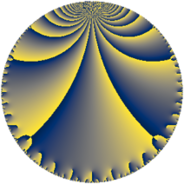# Properties

 Label 76.6.fLevel $76$ Weight $6$ Character orbit 76.f Rep. character $\chi_{76}(27,\cdot)$ Character field $\Q(\zeta_{6})$ Dimension $96$ Newform subspaces $1$ Sturm bound $60$ Trace bound $0$

# Learn more

## Defining parameters

 Level: $$N$$ $$=$$ $$76 = 2^{2} \cdot 19$$ Weight: $$k$$ $$=$$ $$6$$ Character orbit: $$[\chi]$$ $$=$$ 76.f (of order $$6$$ and degree $$2$$) Character conductor: $$\operatorname{cond}(\chi)$$ $$=$$ $$76$$ Character field: $$\Q(\zeta_{6})$$ Newform subspaces: $$1$$ Sturm bound: $$60$$ Trace bound: $$0$$

## Dimensions

The following table gives the dimensions of various subspaces of $$M_{6}(76, [\chi])$$.

Total New Old
Modular forms 104 104 0
Cusp forms 96 96 0
Eisenstein series 8 8 0

## Trace form

 $$96q - 3q^{2} - 11q^{4} - 2q^{5} - 27q^{6} - 3588q^{9} + O(q^{10})$$ $$96q - 3q^{2} - 11q^{4} - 2q^{5} - 27q^{6} - 3588q^{9} + 648q^{10} - 354q^{13} + 2154q^{14} - 611q^{16} - 1006q^{17} + 10524q^{20} + 7824q^{21} + 93q^{22} - 5311q^{24} - 25002q^{25} - 13428q^{26} + 1556q^{28} - 6q^{29} - 12656q^{30} - 30453q^{32} + 8586q^{33} + 30102q^{34} - 2306q^{36} + 29846q^{38} - 1392q^{40} + 29064q^{41} + 18616q^{42} + 9155q^{44} + 13464q^{45} + 38217q^{48} - 116224q^{49} + 36858q^{52} + 26262q^{53} - 26355q^{54} + 53974q^{57} - 112740q^{58} - 108810q^{60} - 77370q^{61} - 106848q^{62} + 337966q^{64} + 166855q^{66} + 175796q^{68} + 148122q^{70} - 259842q^{72} - 52040q^{73} + 103690q^{74} - 194709q^{76} + 197584q^{77} + 253206q^{78} - 171934q^{80} - 313100q^{81} + 63919q^{82} - 161762q^{85} - 38442q^{86} + 10482q^{89} - 372450q^{90} + 64544q^{92} + 94012q^{93} + 537614q^{96} + 177180q^{97} - 509397q^{98} + O(q^{100})$$

## Decomposition of $$S_{6}^{\mathrm{new}}(76, [\chi])$$ into newform subspaces

Label Dim. $$A$$ Field CM Traces $q$-expansion
$$a_2$$ $$a_3$$ $$a_5$$ $$a_7$$
76.6.f.a $$96$$ $$12.189$$ None $$-3$$ $$0$$ $$-2$$ $$0$$